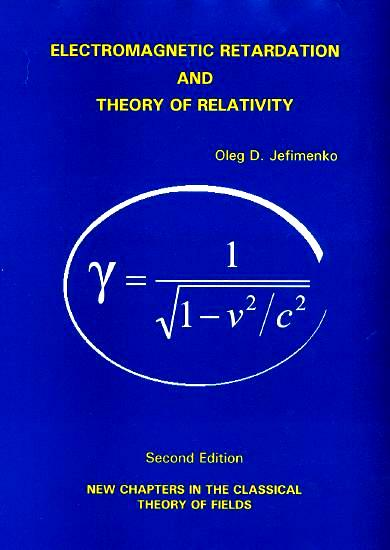# Electromagnetic Retardation and Theory of Relativity

### Hardback - List Price US\$ 44.00 ISBN 0-917406-25-7

Hardback is sold out. Please order the paperback.

CorrectionsThis book presents a comprehensive exposition of the theory of electromagnetic retardation and offers a significant novel approach to the formulation, development and use of the theory of special relativity. The book is divided into two parts. The first part, Chapters 1 to 5, presents the fundamentals of the theory of electromagnetic retardation with emphasis on recently developed electromagnetic relations and mathematical techniques. Employing as the starting point the retarded electromagnetic field integrals rather than the traditional Lienard-Wiechert potentials and using the newest mathematical methods for operations with retarded integrals, the theory is presented in a clear and logical manner, and the applications of the theory are demonstrated by numerous well-chosen original illustrative examples.

As Professor Jefimenko shows, the theory of electromagnetic retardation leads to, and duplicates, many electromagnetic relations that are customarily considered to constitute consequences of relativistic electrodynamics. Much of the first part of the book is devoted to establishing a bridge between the theory of electromagnetic retardation and the theory of relativity. In the second part of the book, Chapters 6 to 11, all the fundamental equations of the special relativity theory, including equations of relativistic electrodynamics and mechanics, are derived in a natural and direct way from equations of electromagnetic retardation and from electromagnetic force and energy equations without any postulates, conjectures, or hypotheses. As a result, the theory of special relativity acquires a new physical and mathematical base and becomes united with Maxwellian electromagnetism into one simple, clear, and harmonious theory of electromagnetic phenomena and mechanical interactions between rapidly moving bodies. Numerous well-chosen original illustrative examples demonstrate various applications of the relativistic electrodynamics and relativistic mechanics developed in this part of the book.

The new approach to the formulations of the theory of relativity presented in this book makes it necessary to reexamine the conventional interpretation of some of the key aspects of the special relativity theory. One of the most significant results of this reexamination is that, although the idea of Lorentz length contraction played an important part in Einstein's approach to the formulation of the theory of relativity, this idea is not an integral part of the theory of relativity itself. Another equally significant result of this reexamination, based on an analysis of a dozen elementary electromagnetic clocks, is that the rate of the moving clocks depends both on the velocity and on the construction of the clocks, so that although all the clocks examined in the book run slow when in motion, only some clocks conform to Einstein's time-dilation formula; others do not.

Finally, the novel approach to the formulation of the special relativity theory developed in this book leads to the conclusion that gravitational phenomena are subject to essentially the same relativistic relations as are the electromagnetic phenomena. Based on this conclusion, a covariant formulation of Newton-Heaviside's gravitational theory is developed and presented in the last chapter of the book.

An Appendix to the book contains an analysis of the physical nature of electric and magnetic forces and presents a novel interpretation of the "near-action" mechanism of electromagnetic interactions.

From the Author. This book is a sequel to my books "Electricity and Magnetism" and "Causality, Electromagnetic Induction, and Gravitation". It is a result of a further exploration of the classical theory of fields in search of heretofore overlooked relations between physical quantities and heretofore overlooked applications of the theory.

As is known, electromagnetic fields propagate with finite velocity. Therefore there always is a time delay before a change in electromagnetic conditions initiated at a point of space produces an effect at any other point of space. This time delay is called electromagnetic retardation. The purpose of this book is to formulate a detailed theory of the phenomenon of electromagnetic retardation and to explore the basic consequences of the theory.

A very significant result of the theory of electromagnetic retardation developed in this book is the derivation of all essential equations of the special relativity theory directly from the fundamental equations of the theory of electromagnetic retardation (retarded electromagnetic field integrals) without any postulates or hypotheses. As a result of this derivation, Maxwellian electrodynamics and the theory of special relativity become united into one simple, clear and harmonious theory of electromagnetic phenomena and of mechanical interactions between rapidly moving bodies.

Although the book presents the results of original research, it is written as a textbook and contains numerous original illustrative examples and derivations demonstrating various applications of the theory developed in the book.